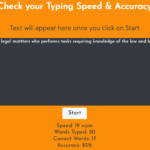## Typing Speed Calculator In JavaScript With Source Code

Typing Speed Calculator is a simple project developed using HTML 5, CSS, and JavaScript. This project helps you to improve your Typing speed. This is like a game, a typing test. Here, you have to copy the provided text exactly in the field given. You can ...

Type : Project

File Size : 1.9 MB# Similar Projects and Reports

#### Typing Speed Calculator In JavaScript With Source Code

Typing Speed Calculator is a simple project developed using HTML 5, CSS, and JavaScript. This project helps you to improve your Typing speed. This is like a game, a typing test. Here, you have to copy the provided text exactly in the field given. You can ...

#### SPEED TYPING IN JAVASCRIPT WITH SOURCE CODE

Speed Typing is a simple project developed using HTML 5, CSS, and JavaScript. This project helps you to improve your Typing speed. This is like a game, just start typing and do not use Backslash to correct your mistakes. Your mistakes will be marked and y...

#### Calculator In JavaScript With Source Code

The calculator is a simple project in HTML5, CSS, and JavaScript. Here, the calculator functions as that of a real-life simple calculator. This project is for solving the mathematical calculations of numbers. You can see the basic features of the calculat...

#### Tip Calculator In Javascript With Source Code

The Tip Calculator is a simple project developed using JavaScript, CSS, and HTML. This project is an interesting project. The user can calculate the tip amount that they can get from a single bill pay.

#### Typing Speed Game In JavaScript With Source Code

Typing Speed Game is a simple project developed using HTML 5, CSS, and JavaScript. This project helps you to improve your Typing speed. This is like a game, a typing test. Here, you have to copy the provided text exactly in the field given. You can start ...

#### Tips Calculator In JavaScript With Source Code

The Tips Calculator is a simple project developed using JavaScript, CSS, and HTML. This project is an interesting project. The user can calculate the tip amount that they can get from a single bill pay. Also, the user can divide the tips into numbers of p...